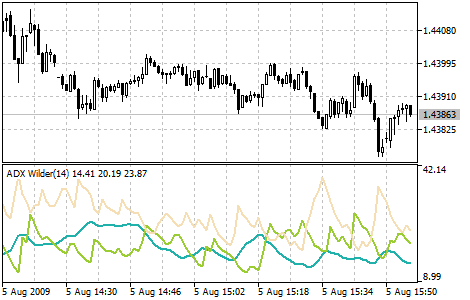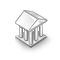• Get access

Interesting script?
So post a link to it -
let others appraise it

You liked the script? Try it in the MetaTrader 5 terminal# Average Directional Movement Index Wilder - indicator for MetaTrader 5

Views:
11855
Rating:
Published:
2010.01.08 12:02
Updated:
2016.11.22 07:32

Average Directional Movement Index Wilder (ADX Wilder) helps to determine if there is a market trend.

This technical indicator is constructed as a strict correspondence with the algorithm described by Welles Wilder in his book "New concepts in technical trading systems".

Trading rules of this indicator are described in the Average Directional Movement Index.Calculation:

First positive (dm_plus) and negative (dm_minus) changes at each bar are calculated, as well as the true range tr:

If High(i) - High(i-1) > 0  dm_plus(i) = High[(i) - High(i-1), otherwise dm_plus(i) = 0.

If Low(i-1) - Low(i) > 0  dm_minus(i) = Low(i-1) - Low(i), otherwise dm_minus(i) = 0.

tr(i) = Max(ABS(High(i) - Low(i)), ABS(High(i) - Close(i-1)), ABS(Low(i) - Close(i-1)))

where:

• High(i) - maximum price of the current bar;
• Low(i) - minimum price of the current bar;
• High(i-1) - maximum price of the previous bar;
• Low(i-1) - minimum price of the previous bar;
• Close(i-1) - close price of the previous bar;
• Max (a, b , c) - maximum value out of three numbers: a, b и c;
• ABS(X)  - absolute value of the X.

After that the smoothed values are calculated: Plus_D(i), Minus_D(i) and ATR():

where:

• SMMA(X, N, i) - Smoothed Moving Average of X series on the current bar;
• Perod_ADX - number of bars, used for calculation.

Now Directional Movement Index - DX(i) - is calculated:

DX(i) = ABS(Plus_D(i) - Minus_D(i))/(Plus_D(i) + Minus_D(i)) * 100

After preliminary calculations we obtain the value of the ADX(i) indicator on the current bar by smoothing DX index values:

Translated from Russian by MetaQuotes Software Corp.
Original code: https://www.mql5.com/ru/code/8Class CalcFrac - Number of bars before and after the current High / Low (calculated fractal)

Calculates fractals and lets you specify the number of bars separately before and after the current High / Low (fractal).Average Directional Movement Index (ADX)

The Average Directional Movement Index Indicator (ADX) helps to determine if there is a price trend.Adaptive Moving Average (AMA)

The Adaptive Moving Average Indicator is used for constructing a moving average with low sensitivity to price series noises and is characterized by the minimal lag for trend detection.Accumulation Swing Index (ASI)

ASI was created by Wales Wilder as an ordinary fluctuations indicator that gets signals from previous maximums and minimums of price.